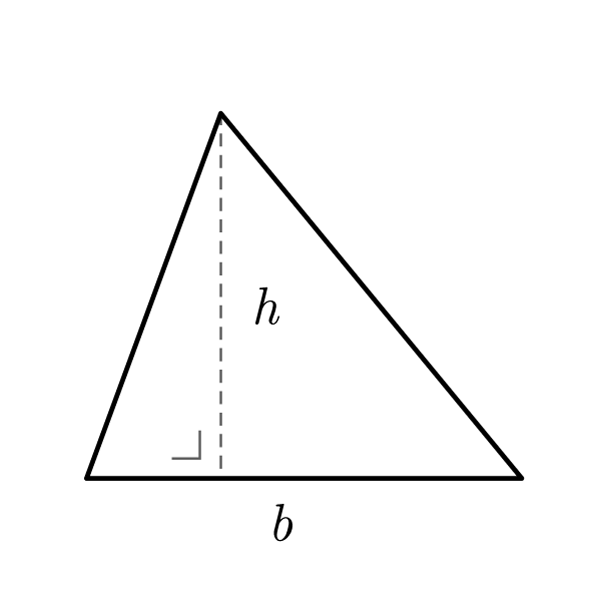# Generic Triangle

 Base $$b$$ Height $$h$$ Side $${S}_{1}$$ Side $${S}_{2}$$ Side $${S}_{3}$$ Perimeter $$2p$$ Semiperimeter $$p$$
$$2p = {S}_{1} + {S}_{2} + {S}_{3}$$
Perimeter
$$A = \frac{b \times h}{2}$$
Area
$$b = \frac{A \times 2}{h}$$
$$h = \frac{A \times 2}{b}$$
$$A = \sqrt{p \times (p - {S}_{1}) \times (p - {S}_{2}) \times (p - {S}_{3}) }$$
Heron's Formula

## Definition

A triangle is a polygon with three sides.

## Properties

1. Generic Triangle's formulas are valid for any triangles## Generic Triangle Formulas

Data Formula
Perimeter 2p = S1 + S2 + S3
Area A = (b × h) / 2
Base b = (A × 2) / h
Height h = (A × 2) / b
Heron's Formula A = √[ p × (p - S1) × (p - S2) × (p - S3) ]